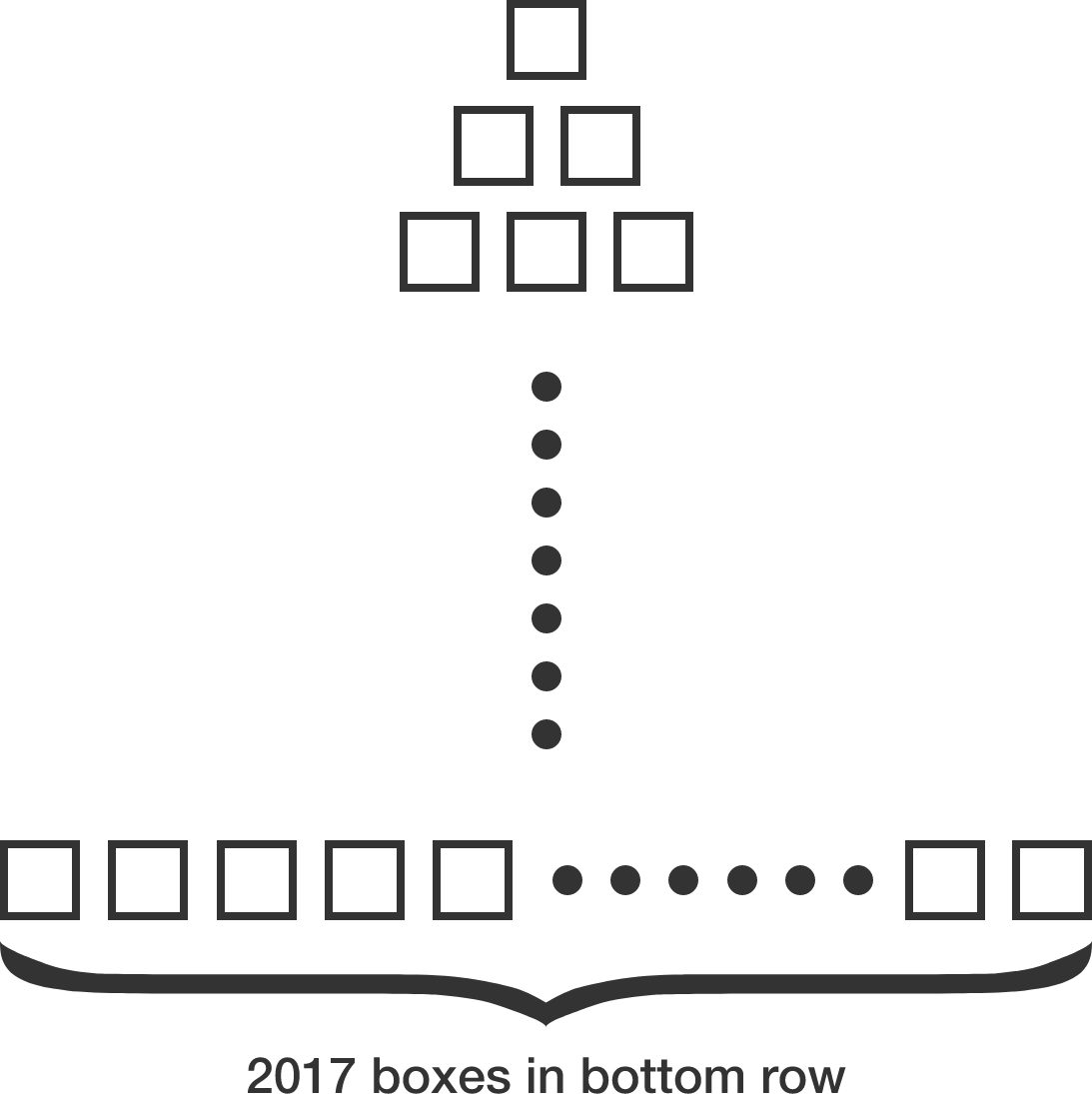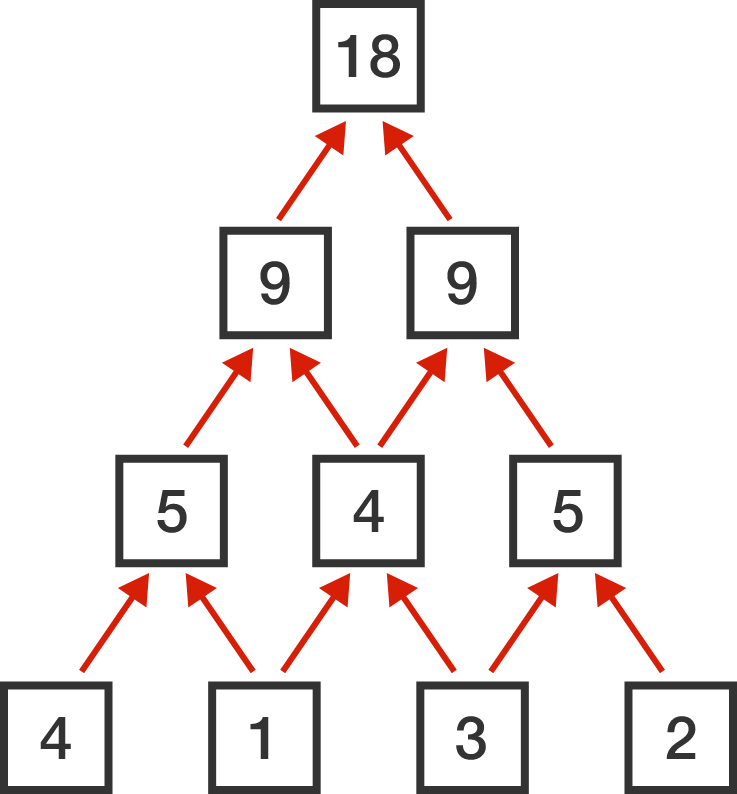# Pyramid Scheme

Probability Level 3

Consider a pyramid with 2017 rows made up of empty boxes. The first (top) row has 1 box, and each successive row has an additional box so that the $2017^\text{th},$ bottom row has 2017 boxes.First, place each of the first 2017 positive integers into the boxes in the bottom row. Then, each empty box in the $2016^\text{th}$ row is filled with the sum of the two numbers beneath it. Then, each successively higher row of boxes is filled in the same way.

Let $M$ and $m$ be the maximum and minimum possible values, respectively, of the single box on the top. Then $M + m = a \times 2^b,$ where $a$ and $b$ are positive integers such that $b$ is as large as possible. Find the value of $a + b.$

Note: Below is an example of how a pyramid of 4 rows could be filled.×